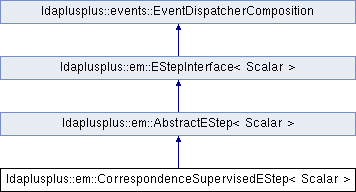LDA++
ldaplusplus::em::CorrespondenceSupervisedEStep< Scalar > Class Template Reference

#include <CorrespondenceSupervisedEStep.hpp>

Inheritance diagram for ldaplusplus::em::CorrespondenceSupervisedEStep< Scalar >:## Public Member Functions

CorrespondenceSupervisedEStep (size_t e_step_iterations=10, Scalar e_step_tolerance=1e-2, Scalar mu=2., Scalar compute_likelihood=1.0, int random_state=0)

std::shared_ptr< parameters::Parametersdoc_e_step (const std::shared_ptr< corpus::Document > doc, const std::shared_ptr< parameters::Parameters > parameters) overridePublic Member Functions inherited from ldaplusplus::em::AbstractEStep< Scalar >
AbstractEStep (int random_state)

virtual void e_step () overridePublic Member Functions inherited from ldaplusplus::events::EventDispatcherComposition
std::shared_ptr< EventDispatcherInterfaceget_event_dispatcher ()

void set_event_dispatcher (std::shared_ptr< EventDispatcherInterface > dispatcher)Protected Member Functions inherited from ldaplusplus::em::AbstractEStep< Scalar >
bool converged (const Eigen::Matrix< Scalar, Eigen::Dynamic, 1 > &gamma_old, const Eigen::Matrix< Scalar, Eigen::Dynamic, 1 > &gamma, Scalar tolerance)

PRNGget_prng ()

## Detailed Description

### template<typename Scalar> class ldaplusplus::em::CorrespondenceSupervisedEStep< Scalar >

CorrespondenceSupervisedEStep implements the expectation step of a variant of the correspondence LDA model as it was introduced in . Iinstead of trying to generate labels it tries to generate the class of the document.

The generative process according to this model is summarized below:

1. Given $$K$$ $$V$$-dimensional multinomial distributions as the topics ( $$\beta$$) and $$K$$ $$C$$-dimensional multinomial distributions ( $$\eta$$) to sample the class labels from.
2. For each of the D documents:
1. Sample from a Dirichlet distribution with Dirichlet prior $$\alpha$$ and create the topic distribution for document d, $$\theta_d \sim Dir\left(\alpha\right)$$.
2. For each of the $$N$$ words $$w_n$$:
1. Sample a topic $$z_n \sim Mult\left( \theta \right)$$
2. From that topic sample a word using the $$k$$th $$V$$-dimensional multinomial distribution, namely $$w_n \sim p(w \mid z_n, \beta)$$
3. From a uniform distribution sample a number $$n$$ between $$1$$ and $$N$$, namely $$\lambda_d \sim Unif\left(1...N\right)$$.
4. Sample a class label for the $$d$$ document from a multinomial distribution, namely $$y_d \sim p\left( y \mid \lambda, z, \eta \right)$$.

Exact probabilistic inference for this model is intractable, thus we use variational inference methods. The factorized distribution on the latent variables is the following.

$$q\left(\theta, z, \lambda \right) = q\left(\theta \mid \gamma\right)\left( \prod_{n=1}^N q\left(z_n \mid \phi_n \right)\right)q\left(\lambda \mid \tau \right)$$

 Blei, D.M. and Jordan, M.I., 2003, July. Modeling annotated data. In Proceedings of the 26th annual international ACM SIGIR conference on Research and development in informaion retrieval (pp. 127-134). ACM.

## Constructor & Destructor Documentation

template<typename Scalar >
 ldaplusplus::em::CorrespondenceSupervisedEStep< Scalar >::CorrespondenceSupervisedEStep ( size_t e_step_iterations = 10, Scalar e_step_tolerance = 1e-2, Scalar mu = 2., Scalar compute_likelihood = 1.0, int random_state = 0 )
Parameters
 e_step_iterations The max number of times to alternate between maximizing for $$\gamma$$ and for $$\phi$$. e_step_tolerance The minimum relative change in the variational parameter $$\gamma$$. mu The uniform Dirichlet prior of $$\eta$$, practically is a smoothing parameter during the maximization of $$\eta$$. compute_likelihood The percentage of documents to compute likelihood for (1.0 means compute for every document) random_state An initial seed value for any random numbers needed

## Member Function Documentation

template<typename Scalar >
 std::shared_ptr< parameters::Parameters > ldaplusplus::em::CorrespondenceSupervisedEStep< Scalar >::doc_e_step ( const std::shared_ptr< corpus::Document > doc, const std::shared_ptr< parameters::Parameters > parameters )
overridevirtual

Maximize the ELBO w.r.t. $$\phi$$ and $$\gamma$$.

The following steps are the mathematics that are implemented where $$\beta$$ are the over words topics distributions, $$\alpha$$ is the Dirichlet prior, $$\eta$$ are the logistic regression parameters, $$\tau$$ are the $$N$$-dimensional mutltinomial parameters, $$i$$ is the topic subscript, $$n$$ is the word subscript, $$\hat{y}$$ is the class subscript, $$y$$ is the document's class, $$w_n$$ is n-th word vocabulary index, and finally $$\Psi(\cdot)$$ is the first derivative of the $$\log \Gamma$$ function.

1. Repeat until convergence of $$\gamma$$.
2. Compute $$\phi_{ni} \propto \beta_{iw_n} \exp\left( \Psi(\gamma_i) + \tau_{ni} * \log\left( \eta_{yi} \right) \right)$$
3. Compute $$\tau_{ni} \propto \exp\left( \sum_{i=1}^K \phi_{ni} * \log\left( \eta_{yi}\right)\right)$$
4. Compute $$\gamma_i = \alpha_i + \sum_n^N \phi_{ni}$$
Parameters
 doc A single document. parameters An instance of class Parameters, which contains all necessary model parameters for e-step's implementation.
Returns
The variational parameters for the current model, after e-step is completed.

Implements ldaplusplus::em::EStepInterface< Scalar >.

The documentation for this class was generated from the following files: